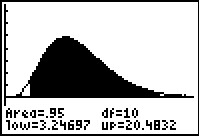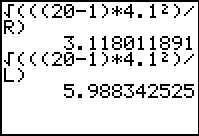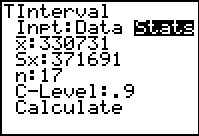••• ##### Device
• TI-83 Plus Family
• TI-84 Plus
• TI-84 Plus Silver Edition
•TI-84 Plus C Silver Edition
•TI-84 Plus CE
• ##### Software

TI Connect™
TI Connect™ CE

• ##### Report an Issue

Statistics: How Far Am I Off?
by Texas Instruments - Bell Ringer Lesson#### Overview

Students calculate a confidence interval using the chi-square distribution to estimate a population variance.

#### Key Steps

•Students review the chi-square distribution and discuss how the non-symmetric distribution requires them to find two critical values when calculating a confidence interval that estimates the population variance.

They will practice finding the left and right critical values using a chart or the INVERSX2 program and then confirm that 95% of the area under the curve is between those two critical values.

•Then students are to calculate the 95% confidence interval for the population variance given the standard deviation of a random sample.

•Two homework problems are given. Students are asked to find confidence intervals for the standard deviation and the mean of the population. Then they can then calculate the actual population mean and standard deviation and determine if it is in their confidence intervals.